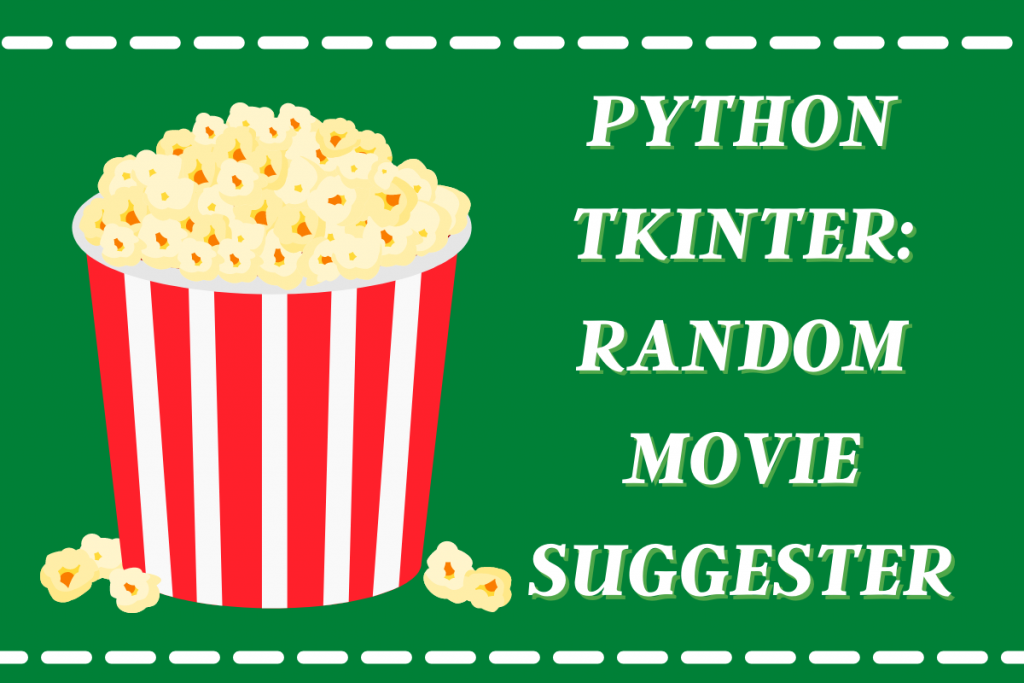# Python Tkinter: Random Movie SuggestionsHey guys! Today in this tutorial we are going to build a simple GUI random movie suggestions app using Python tkinter.

## 1. Data Preparation

To obtain a large dataset containing a lot of movie names we make use of `kaggle`. The dataset we have used in our project can be downloaded from here.

### 1.1 Importing modules

For data preparation we would require two modules namely `numpy` and `pandas`. Along with these modules we will also import `random` and `tkinter` module which we be used in the later section.

The code to import the modules is shown below.

```import numpy as np
import pandas as pd
import random
import tkinter as tk
```

To load the data file of the `csv` format we will use the `read_csv` function of the pandas module and store all the information into a single variable.

To view the data we will be using the `head` function which displays the top 5 rows of the dataset.

The dataset contains 50602 movie names which is obviously a huge number. The code to load the initial data is shown below.

```data = pd.read_csv("indian movies.csv")
```

### 1.3 Data Preparation

Now that the whole data is loaded, we need to observe which columns are needed and which aren’t. The only columns that we require for the project is The Movie Name and the Year in which it was released.

First we convert the whole data into array using numpy module to make the traversal through the data easier. Then we create a empty list to store the necessary information.

The next step involves going through the data row-wise and only storing the movie name and the year in form of a tuple together into a common list.

The code for the same is shown below.

```data = np.array(data)
l_movies=[]
for i in data:
l_movies.append((i,i))
print(l_movies[:5])
```

Let’s print first 5 movie names along with the year using list slicing. The output of the same would be as follows:

```[('Dr. Shaitan', '1960'), ('Nadir Khan', '1968'), ('Apna Sapna Money Money', '2006'), ('Aag Aur Sholay', '1987'), ('Parivar', '1956')]
```

## 2. Creating Tkinter Application Window

The whole window contains Labels, output text boxes and buttons all put together on a single screen. We will also customize the whole window using different colors and fonts.

The code for the whole design process is shown below:

```import tkinter as tk
window = tk.Tk()
window.geometry("600x200")
window.config(bg="#ABEBC6")
window.resizable(width=False,height=False)
window.title('Movie Name Suggestor')

l1 = tk.Label(window,text="Click on the button to suggest you a movie",font=("Arial",20),fg="Black",bg="White")
b1 = tk.Button(window,text="Suggest Movie",font=("Arial",15),bg="darkgreen",fg="white")
t1 = tk.Text(window,width=50,height=1,font=("Arial",15),state='disabled')

l1.place(x=30,y=10)
b1.place(x=200,y=60)
t1.place(x=15,y=120)
window.mainloop()
```

If you have any doubts in the design process, you can refer to the tutorial mentioned here. The final output screen is displayed below.

## 3. Adding Functionality to Button

To add the functionality to the Suggest Movie Button we will be creating a new function which chooses a random movie data from the list we prepared in step 1.

The movie name chosen and year it was released are then added into the output text box. The code for the function is shown below:

```def suggest():
t1.config(state='normal')
t1.delete('1.0', tk.END)
r = random.choice(l_movies)
name = r
year = r
msg = r +"(" + r + ")"
t1.insert(tk.END,msg)
t1.config(state='disabled')
```

After the function is created, all we need to do is add the `command` attribute to the button declaration. And there you go! Your GUI application is complete now!

## Complete code to implement a random movie suggestions app

The complete code for the application is shown below:

```import numpy as np
import pandas as pd
import random
import tkinter as tk

data = np.array(data)
l_movies=[]
for i in data:
l_movies.append((i,i))

def suggest():
t1.config(state='normal')
t1.delete('1.0', tk.END)
r = random.choice(l_movies)
name = r
year = r
msg = r +"(" + r + ")"
t1.insert(tk.END,msg)
t1.config(state='disabled')
window = tk.Tk()
window.geometry("600x200")
window.config(bg="#ABEBC6")
window.resizable(width=False,height=False)
window.title('Movie Name Suggestor')

l1 = tk.Label(window,text="Click on the button to suggest you a movie",font=("Arial",20),fg="Black",bg="White")
b1 = tk.Button(window,text="Suggest Movie",font=("Arial",15),bg="darkgreen",fg="white",command=suggest)
t1 = tk.Text(window,width=50,height=1,font=("Arial",15),state='disabled')

l1.place(x=30,y=10)
b1.place(x=200,y=60)
t1.place(x=15,y=120)
window.mainloop()
```

## Sample Outputs

The images below display the output generated when the user asks the application to get a movie to watch.